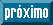## Artigo

•Citado por SciELO
•Acessos

•Similares em SciELO

## versão impressa ISSN 0035-001X

#### Resumo

MARROQUIN, Gustavo; DELGADO, Gerzon E.; GRIMA-GALLARDO, Pedro  e  QUINTERO, Miguel. Preparation, differential thermal analysis and crystal structure of the new quaternary compound CuVInSe3. Rev. mex. fis. [online]. 2018, vol.64, n.6, pp.548-552.  Epub 05-Nov-2019. ISSN 0035-001X.  http://dx.doi.org/10.31349/revmexfis.64.548.

The crystal structure of the quaternary compound CuVInSe ${}_{3}$, belonging to the system (CuInSe ${}_{2}$) ${}_{1-x}$(VSe) ${}_{x}$ with $x=1/2$, was analyzed using X-ray powder diffraction data. This material was synthesized by the melt and anneal method and crystallizes in the tetragonal space group P $\overline{4}$2c (N ${}^{\circ }$ 112), with unit cell parameters $a=5.7909\left(4\right)$ Å, $c=11.625\left(1\right)$ Å, $V=389.84\left(5\right)$ Å${}^{3}$. The Rietveld refinement of 25 instrumental and structural variables led to ${R}_{\text{exp}}=6.6$%, ${R}_{p}=8.7$ %, ${R}_{wp}=8.8$ % and $S=1.3$ for 4501 step intensities, and 153 independent reflections. This compound has a normal adamantane structure and is isostructural with CuFeInSe ${}_{3}$. The DTA indicates that this compound melts at 1332 K.

Palavras-chave : Chemical synthesis; X-ray powder diffraction; crystal structure; differential thermal analysis; chalcogenide; semiconductor; 61.05.cp; 61.50.Nw; 61.66.Fn; 61.40.b.

· texto em Inglês     · Inglês (pdf )